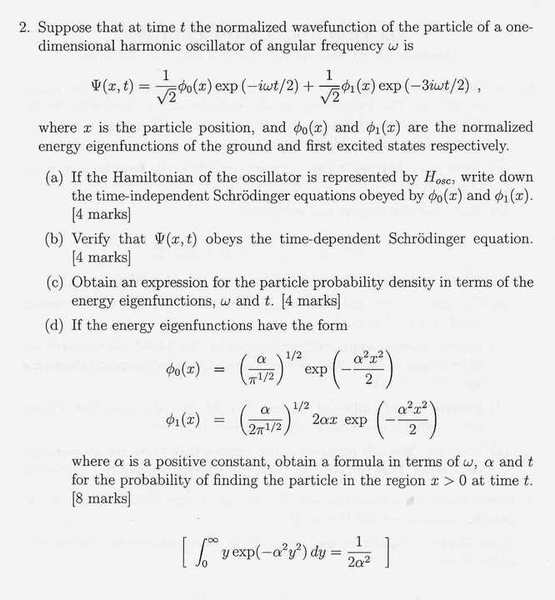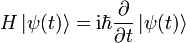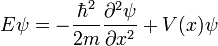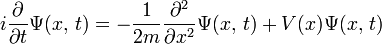# Help with Quantum Mechanics Homework

• andyc10

## Homework Statement

Below is a past paper question from a paper i am working through in preperation for my exams. I am having particular trouble with this question, it doesn't seem as straight forward and formulated as other questions. I am unsure if anything i have done is right so any help would be great.## Homework Equations

(a)Time independent equation(b)
Time dependent Schrodinger(c)
Probabilty Density $$=\left|\psi(x,t)\right|^{2}$$

## The Attempt at a Solution

a)

$$H_{osc}\phi_{0}(x)=E\phi_{0}(x)$$

$$\\\\H_{osc}\phi_{1}(x)=E\phi_{1}(x)$$

b)

I tried simply putting the wavefunction into the Time dependent Schrodinger equation and differentiating. This mustnt be the way since there is an m on the LHS.

c)

Probabilty Density

$$\left|\psi(x,t)\right|^{2}=1/2[\phi^{2}_{0}+\phi^{2}_{1}+\phi^{*}_{0}\phi_{1}exp(-i\omega t)+\phi^{*}_{1}\phi_{0}exp(i\omega t)]$$

d)

$$\int^{\infty}_{0}\left|\psi(x,t)\right|^{2}dx=1/2\int^{\infty}_{0}[\phi^{2}_{0}+\phi^{2}_{1}+\phi^{*}_{0}\phi_{1}exp(-i\omega t)+\phi^{*}_{1}\phi_{0}exp(i\omega t)]dx$$

^ This doesn't work out too well

The purpose of this problem is to show that the given combination of wave functions gives you an oscillating probability density going back and forth at the classical frequency. I don't have time to go through all your equations at the moment but item b, for one, ought to work out the way you've set it up. I think the dimensions are consistent between the LHS and the RHS.

Your time-dependent Schroedinger equation appears to be missing a hbar on the left, and a hbar squared in front of the partial derivative on the right...

Thanks, Matterwave. Good of you to notice that.

I wanted to elaborate on my previous answer a bit: the first three parts of the question are just verifying that you know how to correctly manipulate the formulas for these things, and you seem to be OK. The final part, where you actually have to calculate probabilities, is where you get to see some interesting physics. The combination of states chosen here is actually the very simplest manifestation of a classical type of oscillation using a superposition of quantum states. The quantum states are stationary and have the same likelihood of being anywhere for all time. This particular superposition oscillates back and forth at the classical frequency. What they might have asked you to do is calculate the center of mass of the system, or the x expectation: but they actually ask for something a little different, which is the time spent in the Right Hand Side. Perhaps they're doing you a favor by giving you an easier integration...I'm not sure. But you do appear to have set up the integral correctly. The time-independent terms integrate out to 1/2, because the particle spends half the time in the RHS. The cross terms give you the time dependent oscillation. So you should get the right answer if you can execute the integration...good luck.